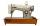# Bar

Half of the bar is green, the third is yellow and the remaining 40cm is red. How long is a bar?

Result

x =  240 cm

#### Solution:

x/2 + x/3 + 40 = x

x = 240

x = 240

Calculated by our simple equation calculator.

Leave us a comment of example and its solution (i.e. if it is still somewhat unclear...):

Showing 0 comments:Be the first to comment!#### To solve this verbal math problem are needed these knowledge from mathematics:

Need help calculate sum, simplify or multiply fractions? Try our fraction calculator. Do you have a linear equation or system of equations and looking for its solution? Or do you have quadratic equation? Do you want to convert length units?

## Next similar examples:

1. Pizza 4Marcus ate half pizza on monday night. He than ate one third of the remaining pizza on Tuesday. Which of the following expressions show how much pizza marcus ate in total?
2. Grandmother and grandfatherGrandmother baked cakes. Grandfather ate half, then quarter of the rest ate Peter and Paul ate half of rest. For parents left 6 cakes. How many cakes maked the grandmother?
3. ClassroomOne-eighth of 9th class was interested in studying at a grammar school, at a business academy one sixth, at secondary vocational schools quarter, to SOU one third and the remaining three students were interested in the school of art direction. How many st
4. Unknown numberI think the number. I'll reduce it to its one-third. The result is then increased by one-third, and I get the number 12.
5. The bunsKate, Zofia and Peter Liked buns. Even today, their grandmother prepare their favorite meal. Katka eats 4 bunches, Žofia 3 and Petra eats 5 buns. Their grandmother said to them, "My inmate will you know how many buns I have been make today, if those you ea
6. Simple equationSolve the following simple equation: 2. (4x + 3) = 2-5. (1-x)
7. Find xSolve: if 2(x-1)=14, then x= (solve an equation with one unknown)
8. Forest nurseryIn the forest nursery after winter, they found that 1/10 stems died out of them. For them, they land 193 new spruces. How many spruces are in the forest nursery?
9. Missing numberBlank +1/6 =3/2 find the missing number
10. Simple equationSolve for x: 3(x + 2) = x - 18
11. Find unknownFind unknown numerator: 4/8 + _/8 = 1
12. Trees3/5 trees are apples, cherries are 1/3. 5 trees are pear. How many is the total number of trees?
13. TailorTailor bought 2 3/4 meters of textile and paid 638 CZK. Determine the price per 1 m of the textile.
14. ZdeněkZdeněk picked up 15 l of water from a 100-liter full-water barrel. Write a fraction of what part of Zdeněk's water he picked.
15. Cupcakes 2Susi has 25 cupcakes. She gives 4/5. How much does she have left?
16. TeacherTeacher Rem bought 360 pieces of cupcakes for the outreach program of their school. 5/9 of the cupcakes were chocolate flavor and 1/4 wete pandan flavor and the rest were vanilla flavor. How much more chocolate flavor cupcakes than vanilla flavor?
17. Mixed2improperWrite the mixed number as an improper fraction. 166 2/3